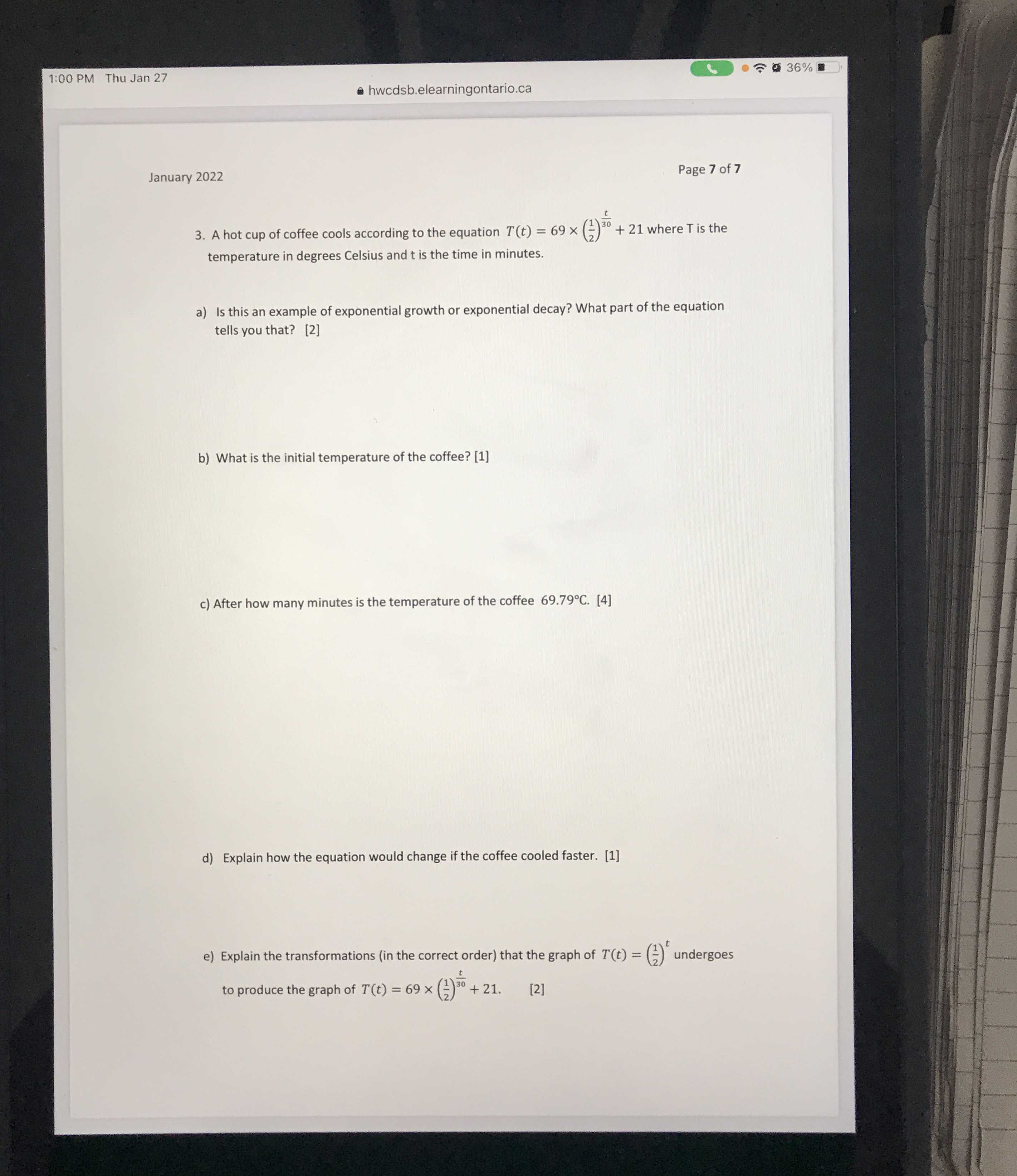### ¿Todavía tienes preguntas de matemáticas?

Pregunte a nuestros tutores expertos
Algebra
Pregunta3. A hot cup of coffee cools according to the equation $$T ( t ) = 69 \times ( \frac { 1 } { 2 } ) ^ { \frac { t } { 30 } } + 21$$ where $$T$$ is the temperature in degrees Celsius and $$t$$ is the time in minutes.

a) Is this an example of exponential growth or exponential decay? What part of the equation tells you that? 

What is the initial temperature of the coffee? 

c) After how many minutes is the temperature of the coffee $$69.79 ^ { \circ } C$$ . 

d) Explain how the equation would change if the coffee cooled faster. 

e) Explain the transformations (in the correct order) that the graph of $$T ( t ) = ( \frac { 1 } { 2 } ) ^ { t }$$ undergoes to produce the graph of $$T ( t ) = 69 \times ( \frac { 1 } { 2 } ) ^ { \frac { t } { 30 } } + 21$$ .

$$T(t)= 69\times 2^{\frac{- t}{30} } + 21$$